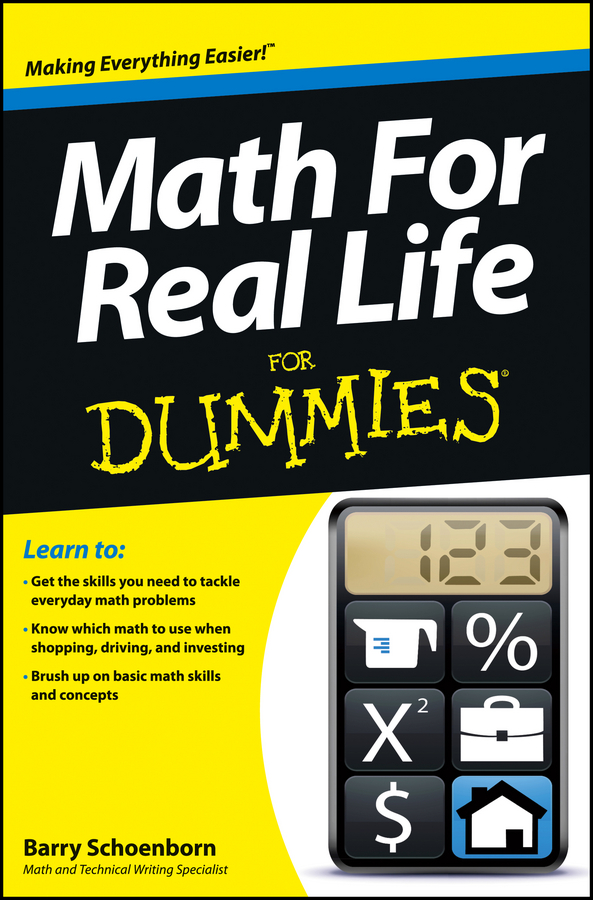##### Math For Real Life For DummiesAnything that can be counted is a unit. Because you can count units, this means that you can apply the Big Four operations (addition, subtraction, multiplication, and division) to them.

Adding and subtracting units isn’t very different from adding and subtracting numbers. Just remember that you can only add or subtract when the units are the same. For example,

3 chairs + 2 chairs = 5 chairs

4 oranges – 1 orange = 3 oranges

What happens when you try to add or subtract different units? Here’s an example:

3 chairs + 2 tables = ?

The only way you can complete this addition is to make the units the same:

3 pieces of furniture + 2 pieces of furniture = 5 pieces of furniture

## Multiply and divide units

You can always multiply and divide units by a number. For example, suppose you have four chairs but find that you need twice as many for a party. Here’s how you represent this idea in math:

4 chairs 2 = 8 chairs

Similarly, suppose you have 20 cherries and want to split them among four people. Here’s how you represent this idea:

20 cherries 4 = 5 cherries

But you have to be careful when multiplying or dividing units by units. For example:

2 apples 3 apples = ? WRONG!

12 hats 6 hats = ? WRONG!

Neither of these equations makes any sense. In these cases, multiplying or dividing by units is meaningless.

In many cases, however, multiplying and dividing units is okay. For example, multiplying units of length (such as inches, miles, or meters) results in square units:

3 inches 3 inches = 9 square inches

10 miles 5 miles = 50 square miles

100 meters 200 meters = 20,000 square meters

Similarly, here are some examples of when dividing units makes sense:

12 slices of pizza 4 people = 3 slices of pizza/person

140 miles 2 hours = 70 miles/hour

In these cases, you read the fraction slash (/) as per: slices of pizza per person or miles per hour.